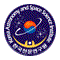# Korean VLBI Network (KVN)

## Beam Alignment

For the simultaneous observations at the 4 frequency bands, the quasi-optics should be aligned so that all the 4 beams point at the same position in the sky. The following table shows pointing offsets of the other frequency bands with respect to the 86GHz LCP beam center. The relative offsets were obtained from the result of Cross Scan after the alignment. As the results, the 22 GHz beam is aligned within 5 arc seconds, while those of 129 and 43 GHz are aligned within 3 arc seconds.

Beam Alignment
Site Band (GHz) Az Offset (arcsec) El Offset (arcsec)
KVNYS 22 L −0.8 (+/−0.4) −0.4 (+/−0.4)
22 R −1.7 (+/−0.4) −0.9 (+/−0.4)
43 L 1.1 (+/−0.3) −0.9 (+/−0.2)
43 R 0.4 (+/−0.4) −1.3 (+/−0.3)
86 L --- ---
86 R 2.2 (+/−0.5) 0.0 (+/−0.6)
129 L −2.8 (+/−0.5) 0.4 (+/−0.6)
129 R −4.0 (+/−0.5) −0.3 (+/−0.8)
KVNUS 22 L −1.9 (+/−0.6) 3.8 (+/−0.8)
22 R −3.1 (+/−0.6) 3.3 (+/−0.8)
43 L −1.4 (+/−0.6) 1.9 (+/−0.7)
43 R −1.9 (+/−0.6) 1.6 (+/−0.7)
86 L --- ---
86 R
129 L 2.4 (+/−1.5) −3.0 (+/−1.6)
129 R
KVNTN 22 L −2.8 (+/−0.7) 2.8 (+/−0.8)
22 R −3.8 (+/−0.6) 3.5 (+/−0.7)
43 L 2.3 (+/−0.3) −0.2 (+/−0.3)
43 R 1.9 (+/−0.4) −0.8 (+/−0.1)
86 L --- ---
86 R 2.2 (+/−0.5) −0.0 (+/−0.4)
129 L 1.9 (+/−1.2) 0.1 (+/−1.2)
129 R −0.2 (+/−1.3) −0.1 (+/−1.2)Korean VLBI Network